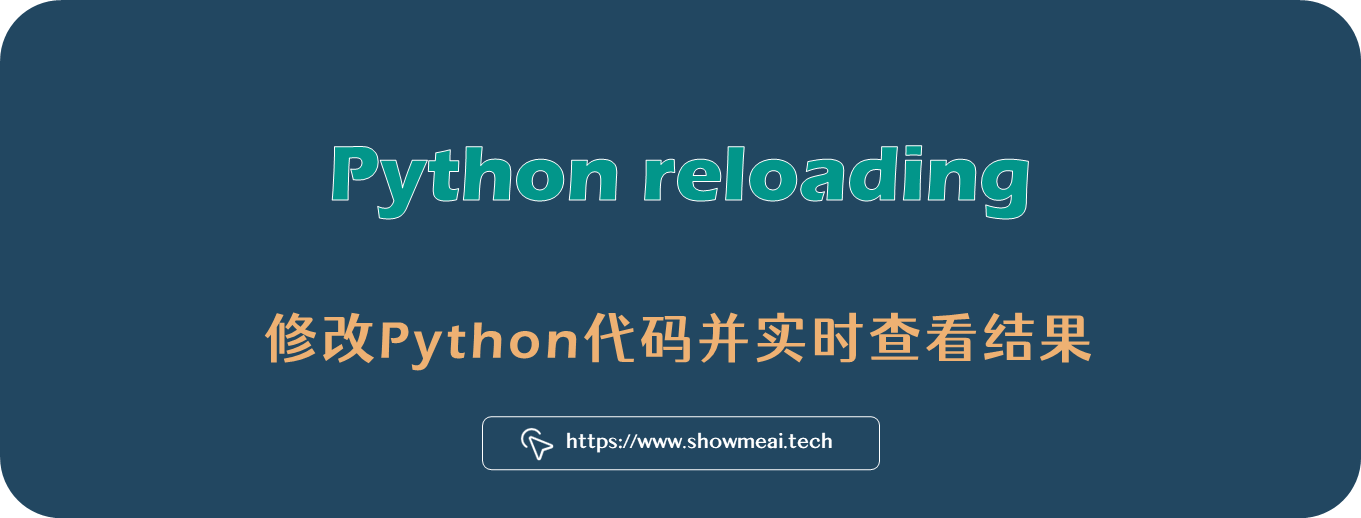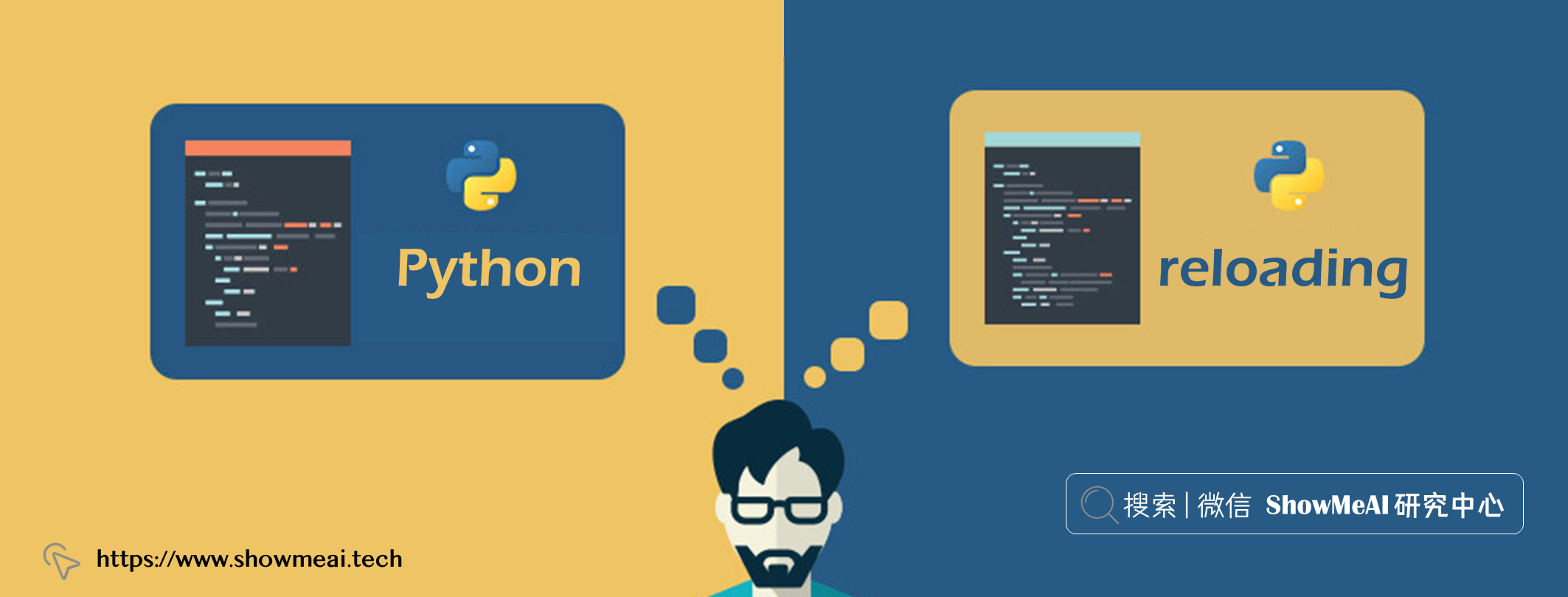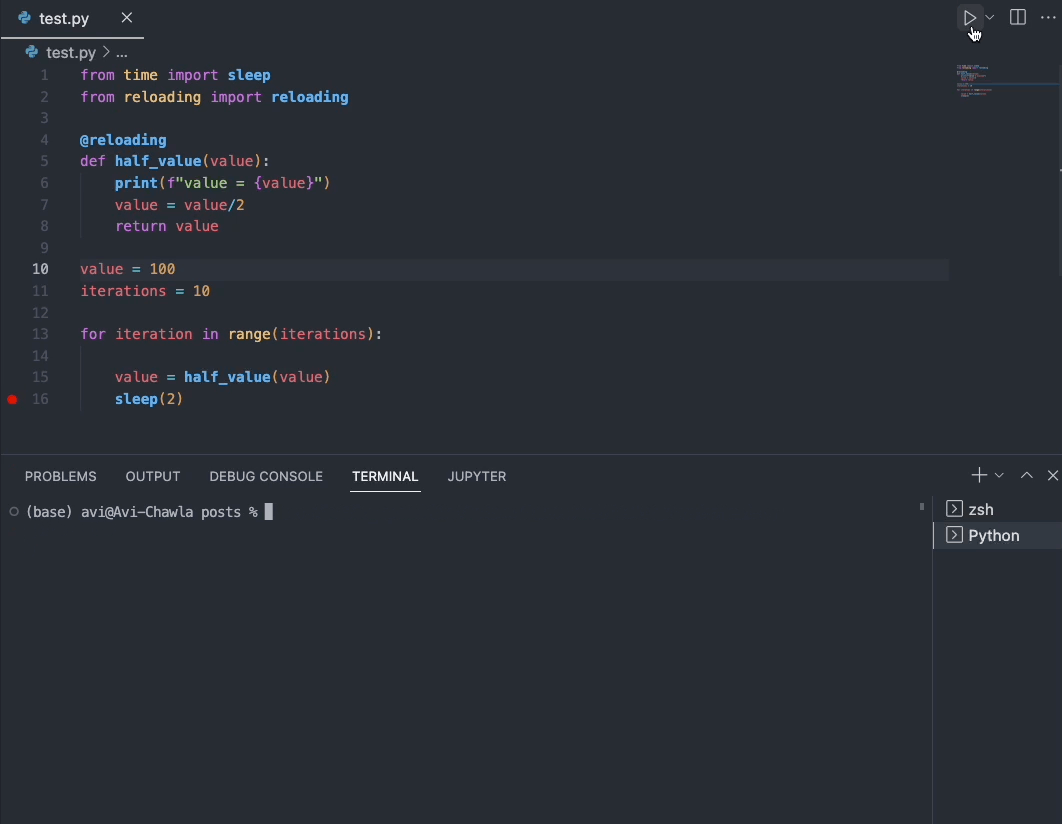# 熱載入技術：修改Python程式碼並實時檢視結果 ⛵# 💡 引言`pip install reloading`

## 💦 重新載入迴圈體程式碼

```python from time import sleep

value = 100 iterations = 10

for iteration in range(iterations):

``````print(f"value = {value}")
value = value/2
sleep(2)
``````

```

value = 100 iterations = 10

``````print(f"value = {value}")
value = value/2
sleep(2)
``````

```## 💦 重新載入修改後的函式

```python from time import sleep

def half_value(value): print(f"value = {value}") value = value/2 return value

value = 100 iterations = 10

for iteration in range(iterations):

``````value = half_value(value)
sleep(2)
``````

```

value = 100 iterations = 10

for iteration in range(iterations):

``````value = half_value(value)
sleep(2)
``````

```# 推薦閱讀